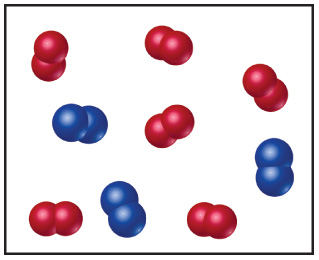# Problem: The following diagram represents the collection of elements formed by a decomposition reaction. If the blue spheres represent N atoms and the red ones represent O atoms, what was the empirical formula of the original compound?Could you draw a diagram representing the molecules of the compound that had been decomposed? Why or why not?

###### FREE Expert Solution

The products of the decomposition reactions are N2 and O2. This suggests that the original compound contained both of these elements.

Recall that the empirical formula is the simplest whole-number ratio formula of a compound

97% (25 ratings)###### Problem Details

The following diagram represents the collection of elements formed by a decomposition reaction.If the blue spheres represent N atoms and the red ones represent O atoms, what was the empirical formula of the original compound?
Could you draw a diagram representing the molecules of the compound that had been decomposed? Why or why not?

Frequently Asked Questions

What scientific concept do you need to know in order to solve this problem?

Our tutors have indicated that to solve this problem you will need to apply the Empirical Formula concept. You can view video lessons to learn Empirical Formula. Or if you need more Empirical Formula practice, you can also practice Empirical Formula practice problems.